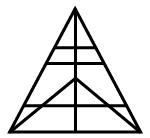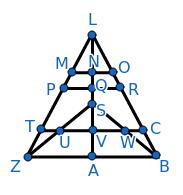Question 3

# How many triangles are there in the given figure?SolutionThe different triangles in the given figure are LNM, LNO, LMO, LQP, LQR, LPR, LVT, LVC, LTC, LAZ, LAB, LZB, SVU, SVW, SUW, SAZ, SAB, SZB, SZL, SBL, TUZ, CBW.

$$\therefore\$$The number of triangles in the given figure is 22.

Hence, the correct answer is Option A

• Free SSC Study Material - 18000 Questions
• 230+ SSC previous papers with solutions PDF